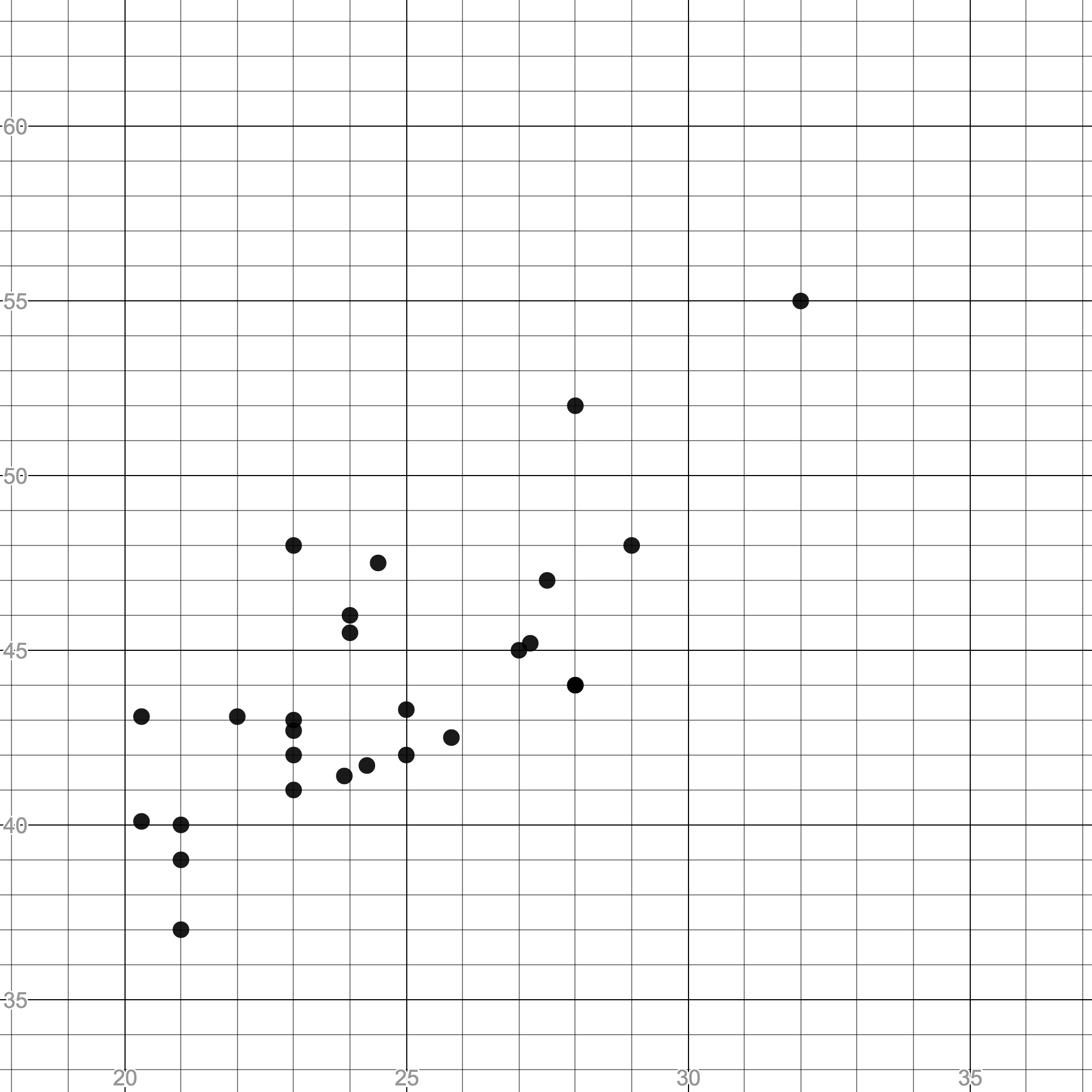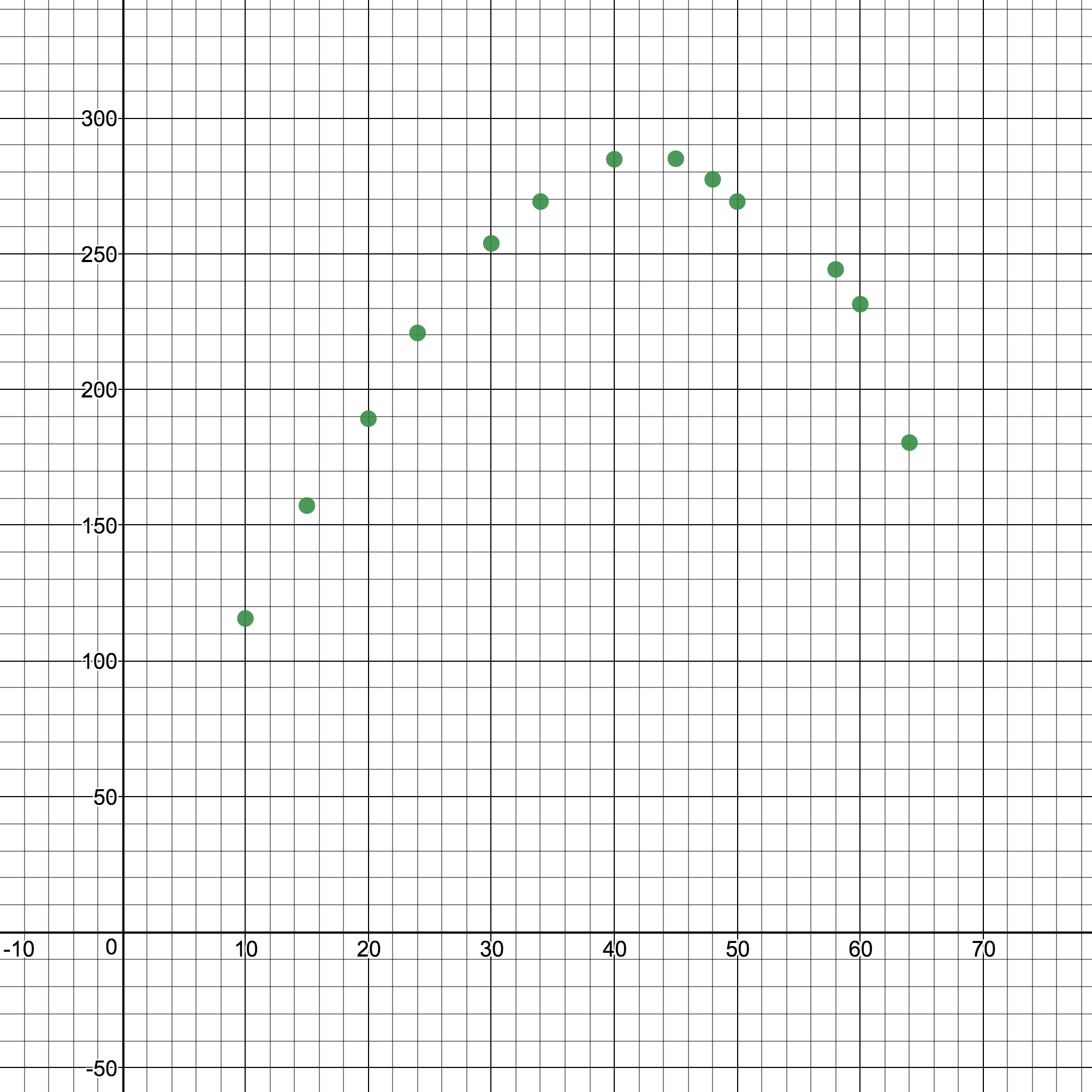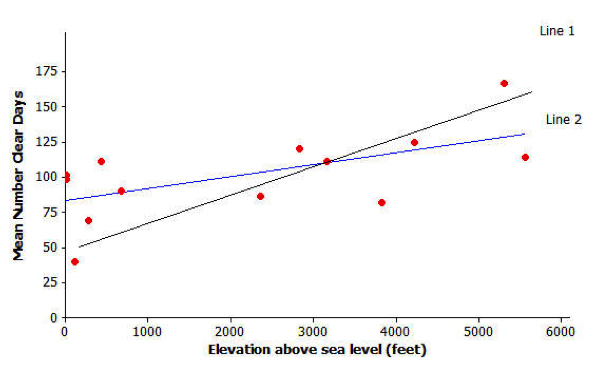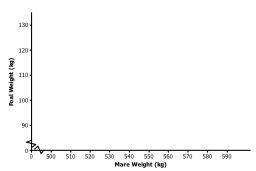# Descriptive Statistics

## Objective

Determine the function of best fit and create a linear equation from least squares regression using technology.

## Common Core Standards

### Core Standards

?

• HSS-ID.B.6a — Fit a function to the data; use functions fitted to data to solve problems in the context of the data. Use given functions or choose a function suggested by the context. Emphasize linear, quadratic, and exponential models.

• HSS-ID.B.6b — Informally assess the fit of a function by plotting and analyzing residuals.

• HSS-ID.C.7 — Interpret the slope (rate of change) and the intercept (constant term) of a linear model in the context of the data.

?

• 8.SP.A.2

• 8.SP.A.3

## Criteria for Success

?

1. Identify the overall shape of the points in the scatterplot and assign a function of best fit.
2. Describe the difference between the actual data (the points on the scatterplot) and the generality or prediction formed by the function of best fit.
3. Generalize the trend of a set of data represented in a scatterplot by estimating a line of best fit and writing an appropriate equation.
4. Interpret the slope (rate of change) and the intercept (constant) in terms of the context being modeled.
5. Explain that the “least squares regression” is a technical term for calculating, rather than estimating, the line of best fit. “Least squares” refers to the use of residuals to determine how “good” your function is.
6. Understand that a regression can be calculated in any function (quadratic regression, exponential regression) to develop an algebraic model that fits the situation well. We refer to this as the “function of best fit.”
7. Use a calculator to identify the slope and intercept for the line of best fit for a set of data.
8. Compare the calculated least squares regression with the estimated line of best fit.

## Tips for Teachers

?

• Students should identify the function of best fit but will not need to do any other regressions besides linear.
• The following resource from, Desmos, “Learn Desmos: Regressions” may be helpful to use as a quick, non-graphing or scientific calculator way to do least squares regression.

## Anchor Problems

?

### Problem 1

Draw a line of best fit for the data shown comparing the arm length in centimeters as a function of the foot length in centimeters. Write the equation of the line of best fit.### Problem 2

Draw a line of best fit. Write the equation of the line.## Problem Set

?

The following resources include problems and activities aligned to the objective of the lesson that can be used to create your own problem set.

• Feel free to extend any of the scatterplots into 8th grade, thinking of line of best fit and writing the equation of the line of best fit. The teacher can also do some other function review with some of the other scatterplots.

?

### Problem 1

The scatterplot below displays the elevation and mean number of clear days per year of  U.S. cities. Two lines are shown on the scatterplot. Which represents the least squares line? Explain your choice.#### References

EngageNY Mathematics Algebra I > Module 2 > Topic D > Lesson 14Exit Ticket #1

Algebra I > Module 2 > Topic D > Lesson 14 of the New York State Common Core Mathematics Curriculum from EngageNY and Great Minds. © 2015 Great Minds. Licensed by EngageNY of the New York State Education Department under the CC BY-NC-SA 3.0 US license. Accessed Dec. 2, 2016, 5:15 p.m..

### Problem 2

1.    Below is a scatterplot of foal birth weight and mare’s weight.1. The equation of the least squares line for the data is ${y=-19.6+0.248x}$ , where x = mare’s weight (in kg) and y = foal’s birth weight (in kg).  What foal birth weight would you predict for a mare that weighs 520 kg?
2. How would you interpret the value of the slope in the least squares line?

#### References

EngageNY Mathematics Algebra I > Module 2 > Topic D > Lesson 14Exit Ticket #2

Algebra I > Module 2 > Topic D > Lesson 14 of the New York State Common Core Mathematics Curriculum from EngageNY and Great Minds. © 2015 Great Minds. Licensed by EngageNY of the New York State Education Department under the CC BY-NC-SA 3.0 US license. Accessed Dec. 2, 2016, 5:15 p.m..